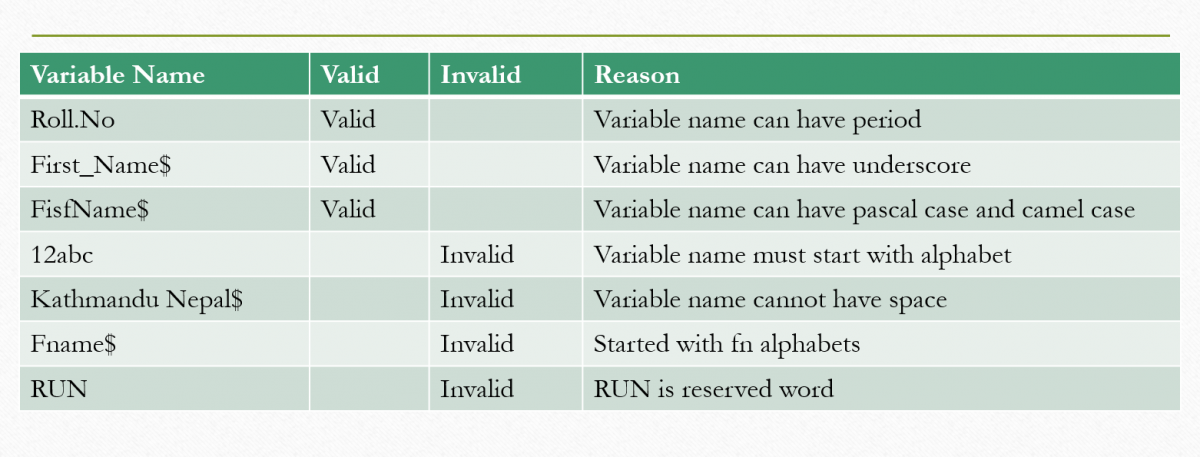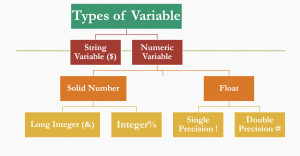# Variables## Variables

A variable is a name of storage location in the computer’s memory, we can store different values in a variable. A variable is needed in the program to store different data in the computer memory which are used for processing. The value stored in the variable is not fixed as its value may change during program execution time.

## Rules for naming a variable

1. A variable beginning should be with alphabets like : a, b, c, or A,B, C
2. We can’t use keyword as a variable
3. We should declare variable with a valid name means a unique name should be given to a variable.
4. Variable name must be only up to 40 characters long in QBASIC.
5. We can only use characters like: #,&,\$,% and ! for declaring variable.
6. Variables names are not case sensitive

## Some valid or invalid Variables

 Variable Name Valid Invalid Reason Roll.No Valid Variable name can have period First_Name\$ Valid Variable name can have underscore FisfName\$ Valid Variable name can have pascal case and camel case 12abc Invalid Variable name must start with alphabet Kathmandu Nepal\$ Invalid Variable name cannot have space Fname\$ Invalid Started with fn alphabets RUN Invalid RUN is reserved word

### There are Two types of variable

1. String Variable
2. Numeric Variable## String Variable

A variable which stores alphanumeric data is known as string variable it occupies 4 bytes for description and one byte for each character for string data.

``````
CLS
PRINT "Welcome to Hi Tech Computer"
name\$ = "Yam Soti"
PRINT name\$
END
``````

### Numerical Variable

A variable which stores only number (numeric data) is called numeric variable. Numeric data means that can perform mathematical calculations.

Types of Numeric Variable

1. Integer
2. Long Integer
3. Single Precision
4. Double Precision

1. Integer Variable

The integer variable stores a whole numbers without decimals. Integer may range from -32768 to 32767. It occupies 2 byte of memory to store an integer. Ex. X% = 15, RollNumber%, Age% etc.

``````
CLS
a% = 12
b% = 10
sum = a%+b%
Print sum
End
``````

2. Long Integer Variable

The long integer variable can store a large range of whole number without decimals. It stores long integer ranges from -2147483648 to 2147483647. It occupies 4 byte in memory to store long integer. Ex. acNumber&, registrationNumber&  etc.

``````
CLS
a& = 12345789
b& = 10542
sum = a&+b&
Print sum
End
``````

3. Single Precision Variable

Single precision variable stores whole number with decimal point. It stores up to 7 digits number. It occupies 4 byte in memory to store numeric data. Ex. BasicSalary!, price!, tax! Etc.

Example:

``````
CLS
salary! = 9500.50
bonus! = 1296.81
sum = salary! + bonus!
Print sum
End
``````

4. Double Precision Variable

Double precision variable also stores whole number as well as number decimal point. It stores number having up to 16 digits. It occupies 8 byte in memory to store number. Ex. lightSpeed#, distanceMoon# etc.

Example:

``````
CLS
abc# =123456789.50
D# = 123456789D+10
Print abc#
Print D#
End
``````